# Existence of solutions for nonlocal p-Laplacian thermistor problems on time scales

## Abstract

In this paper, a nonlocal initial value problem to a p-Laplacian equation on time scales is studied. The existence of solutions for such a problem is obtained by using the topological degree method.

## 1 Introduction

In this paper, we are concerned with the existence of solutions of the following nonlocal p-Laplacian dynamic equation on a time scale $\mathbb{T}$:

$-{\left({\varphi }_{p}\left({u}^{\mathrm{△}}\left(t\right)\right)\right)}^{\mathrm{\nabla }}=\frac{\lambda a\left(t\right)f\left(u\left(t\right)\right)}{{\left({\int }_{0}^{T}f\left(u\left(s\right)\right)\mathrm{\nabla }s\right)}^{k}},\phantom{\rule{1em}{0ex}}\mathrm{\forall }t\in {\left(0,T\right)}_{\mathbb{T}},$
(1.1)

with integral initial value

$\begin{array}{r}u\left(0\right)={\int }_{0}^{T}g\left(s\right)u\left(s\right)\mathrm{\nabla }s,\\ {u}^{\mathrm{△}}\left(0\right)=A,\end{array}$
(1.2)

where ${\varphi }_{p}\left(\cdot \right)$ is the p-Laplace operator defined by ${\varphi }_{p}\left(s\right)={|s|}^{p-2}s$, $p>1$, ${\varphi }_{p}^{-1}={\varphi }_{q}$ with q the Hölder conjugate of p, i.e., $\frac{1}{p}+\frac{1}{q}=1$, $\lambda >0$, $k>0$, $f:{\left[0,T\right]}_{\mathbb{T}}⟶{\mathbb{R}}^{+\ast }$ is continuous (${\mathbb{R}}^{+\ast }$ denotes positive real numbers), $a:{\left[0,T\right]}_{\mathbb{T}}⟶{\mathbb{R}}^{+}$ is left dense continuous, $g\left(s\right)\in {L}^{1}\left({\left[0,T\right]}_{\mathbb{T}}\right)$ and A is a real constant.

This model arises in ohmic heating phenomena, which occur in shear bands of metals which are deformed at high strain rates [1, 2], in the theory of gravitational equilibrium of polytropic stars , in the investigation of the fully turbulent behavior of real flows, using invariant measures for the Euler equation , in modeling aggregation of cells via interaction with a chemical substance (chemotaxis) . For the one-dimensional case, problems with the nonlocal initial condition appear in the investigation of diffusion phenomena for a small amount of gas in a transparent tube [6, 7]; nonlocal initial value problems in higher dimension are important from the point of view of their practical applications to modeling and investigating of pollution processes in rivers and seas, which are caused by sew-age .

The study of dynamic equations on time scales has led to some important applications , and an amount of literature has been devoted to the study the existence of solutions of second-order nonlinear boundary value problems (e.g., see ).

Motivated by the above works, in this paper, we study the existence of solutions to Problem (1.1), (1.2). Compared with the works mentioned above, this article has the following new features: firstly, the main technique used in this paper is the topological degree method; secondly, Problem (1.1), (1.2) involves the integral initial condition.

The paper is organized as follows. We introduce some necessary definitions and lemmas in the rest of this section. In Section 2, we provide some necessary preliminaries, and in Section 3, the main results are stated and proved.

Definition 1.1 For $t and $r>inf\mathbb{T}$, define the forward jump operator σ and the backward jump operator ρ, respectively,

$\sigma \left(t\right)=inf\left\{\tau \in \mathbb{T}\mid \tau >t\right\}\in \mathbb{T},\phantom{\rule{2em}{0ex}}\rho \left(r\right)=sup\left\{\tau \in \mathbb{T}\mid \tau

for all $t,r\in \mathbb{T}$. If $\sigma \left(t\right)>t$, t is said to be right scattered, and if $\rho \left(r\right), r is said to be left scattered. If $\sigma \left(t\right)=t$, t is said to be right dense, and if $\rho \left(r\right)=r$, r is said to be left dense. If $\mathbb{T}$ has a right scattered minimum m, define; otherwise, set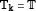. If $\mathbb{T}$ has a left scattered maximum M, define; otherwise, set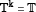.

Definition 1.2 For $x:\mathbb{T}⟶\mathbb{R}$ and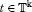, we define the delta derivative of $x\left(t\right)$, ${x}^{\mathrm{△}}\left(t\right)$, to be the number (when it exists) with the property that for any $\epsilon >0$, there is a neighborhood U of t such that

$|\left[x\left(\sigma \left(t\right)\right)-x\left(s\right)\right]-{x}^{\mathrm{△}}\left(t\right)\left[\sigma \left(t\right)-s\right]|<\epsilon |\sigma \left(t\right)-s|$

for all $s\in U$. For $x:\mathbb{T}⟶\mathbb{R}$ and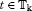, we define the nabla derivative of $x\left(t\right)$, ${x}^{\mathrm{\nabla }}\left(t\right)$, to be the number (when it exists) with the property that for any $\epsilon >0$, there is a neighborhood V of t such that

$|\left[x\left(\rho \left(t\right)\right)-x\left(s\right)\right]-{x}^{\mathrm{\nabla }}\left(t\right)\left[\rho \left(t\right)-s\right]|<\epsilon |\rho \left(t\right)-s|$

for all $s\in V$.

Definition 1.3 If ${F}^{\mathrm{△}}\left(t\right)=f\left(t\right)$, then we define the delta integral by

${\int }_{a}^{t}f\left(s\right)\mathrm{△}s=F\left(t\right)-F\left(a\right).$

If ${\mathrm{\Phi }}^{\mathrm{\nabla }}\left(t\right)=f\left(t\right)$, then we define the nabla integral by

${\int }_{a}^{t}f\left(s\right)\mathrm{\nabla }s=\mathrm{\Phi }\left(t\right)-\mathrm{\Phi }\left(a\right).$

Throughout this paper, we assume that $\mathbb{T}$ is a nonempty closed subset of with $0\in {\mathbb{T}}_{k}$, $T\in {\mathbb{T}}^{k}$.

Lemma 1.1 (Alternative theorem)

Suppose that X is a Banach space and A is a completely continuous operator from X to X. Then for any $\lambda \ne 0$, only one of the following statements holds:

1. (i)

For any $y\in X$, there exists a unique $x\in X$, such that

$\left(A-\lambda I\right)x=y;$
2. (ii)

There exists an $x\in X$, $x\ne 0$, such that

$\left(A-\lambda I\right)x=0.$

## 2 Preliminaries

Let $E={C}_{ld}\left({\left[0,T\right]}_{T},R\right)$ be a Banach space equipped with the maximum norm $\parallel u\parallel =max{lim}_{{\left[0,T\right]}_{T}}|u\left(t\right)|$.

Consider the following problem: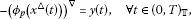(2.1)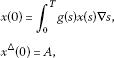(2.2)

where $y\in C\left({\left[0,T\right]}_{\mathbb{T}}\right)$, ${\int }_{0}^{T}g\left(s\right)\mathrm{\nabla }s\ne 1$.

Integrating Eq. (2.1) from 0 to t, one obtains

${\varphi }_{p}\left({x}^{\mathrm{△}}\left(t\right)\right)-{\varphi }_{p}\left({x}^{\mathrm{△}}\left(0\right)\right)=-{\int }_{0}^{t}y\left(s\right)\mathrm{\nabla }s.$

Using the initial condition (2.2), we have

${x}^{\mathrm{△}}\left(t\right)={\varphi }_{p}^{-1}\left({\varphi }_{p}\left(A\right)-{\int }_{0}^{t}y\left(s\right)\mathrm{\nabla }s\right).$

Integrating the above equality from 0 to t again, we obtain

$x\left(t\right)-{\int }_{0}^{T}g\left(s\right)x\left(s\right)\mathrm{\nabla }s={\int }_{0}^{t}{\varphi }_{p}^{-1}\left({\varphi }_{p}\left(A\right)-{\int }_{0}^{\tau }y\left(s\right)\mathrm{\nabla }s\right)\mathrm{△}\tau .$
(2.3)

Let $F\left(t\right):={\int }_{0}^{t}{\varphi }_{p}^{-1}\left({\varphi }_{p}\left(A\right)-{\int }_{0}^{\tau }y\left(s\right)\mathrm{\nabla }s\right)\mathrm{△}\tau$.

Define an operator $K:{C}_{ld}\left({\left[0,T\right]}_{\mathbb{T}}\right)⟶{C}_{ld}\left({\left[0,T\right]}_{\mathbb{T}}\right)$ by

$\left(Kx\right)={\int }_{0}^{T}g\left(s\right)x\left(s\right)\mathrm{\nabla }s,$

then (2.3) can be rewritten as

$\left(I-K\right)x\left(t\right)=F\left(t\right).$
(2.4)

Thus, $x\left(t\right)$ is a solution to (2.1), (2.2) if and only if it is a solution to (2.4).

Lemma 2.1 $I-K$ is a Fredholm operator.

Proof To prove that $I-K$ is a Fredholm operator, we need only to show that K is completely continuous.

It is easy to see from the definition of K that K is a bounded linear operator from ${C}_{ld}\left({\left[0,T\right]}_{\mathbb{T}}\right)$ to ${C}_{ld}\left({\left[0,T\right]}_{\mathbb{T}}\right)$. Obviously, $dimR\left(K\right)=1$. So, K is a completely continuous operator. This completes the proof. □

Lemma 2.2 Problem (2.1), (2.2) admits a unique solution.

Proof Since Problem (2.1), (2.2) is equivalent to Problem (2.4), we need only to show that Problem (2.4) has a unique solution.

Using Lemma 2.1 and the alternative theorem, it is sufficient to prove that

$\left(I-K\right)x\left(t\right)=0$
(2.5)

has a trivial solution $x\equiv 0$ only.

On the contrary, suppose (2.5) has a nontrivial solution μ, then μ is a constant, and we have

$I\mu =K\mu =\mu .$

The definition of K and the above equality yield

$\left[1-{\int }_{0}^{T}g\left(s\right)\mathrm{\nabla }s\right]\mu =0,$

which is a contradiction to the assumptions ${\int }_{0}^{T}g\left(s\right)\mathrm{\nabla }s\ne 1$ and $\mu \not\equiv 0$.

Thus, we complete the proof. □

## 3 Main results

Throughout this section, we assume that the following conditions hold.

(H1) ${\int }_{0}^{T}|g\left(s\right)|\mathrm{\nabla }s=M<1$;

(H2) $f:{\left[0,T\right]}_{\mathbb{T}}⟶{\mathbb{R}}^{+\ast }$ is continuous;

(H3) $a:{\left[0,T\right]}_{\mathbb{T}}⟶{\mathbb{R}}^{+}$ is left dense continuous and ${max}_{t\in {\left[0,T\right]}_{\mathbb{T}}}a\left(t\right)\le {M}_{1}$;

(H4) $f\left(y\right)\le {\left[{c}_{1}{\varphi }_{p}\left(|y|\right)+{c}_{2}\right]}^{\frac{1}{1-k}}$, ${c}_{1},{c}_{2}>0$ and ${c}_{1}<\frac{{\varphi }_{p}\left(\frac{1-M}{{2}^{q-1}T}\right)}{\lambda {M}_{1}{T}^{1-k}}$, when $k<1$;

(H5) $f\left(y\right)\ge {\left[{c}_{3}{\varphi }_{p}\left(|y|\right)\right]}^{\frac{1}{1-k}}$, ${c}_{3}>0$ and ${c}_{3}<\frac{{\varphi }_{p}\left(\frac{1-M}{{2}^{q-1}T}\right)}{\lambda {M}_{1}{T}^{1-k}}$, when $k>1$.

From Lemma 2.2 we know that $u\left(t\right)$ is a solution to Problem (1.1), (1.2) if and only if it is a solution to the following integral equation:

$\left(I-K\right)u\left(t\right)={\int }_{0}^{t}{\varphi }_{p}^{-1}\left({\varphi }_{p}\left(A\right)-{\int }_{0}^{\tau }\frac{\lambda a\left(s\right)f\left(u\left(s\right)\right)}{{\left({\int }_{0}^{T}f\left(u\left(s\right)\right)\mathrm{\nabla }s\right)}^{k}}\mathrm{\nabla }s\right)\mathrm{△}\tau .$
(3.1)

Define an operator $F:{C}_{ld}\left({\left[0,T\right]}_{\mathbb{T}}\right)⟶{C}_{ld}\left({\left[0,T\right]}_{\mathbb{T}}\right)$ by

$\left(Fu\right)\left(t\right)={\int }_{0}^{t}{\varphi }_{p}^{-1}\left({\varphi }_{p}\left(A\right)-{\int }_{0}^{\tau }\frac{\lambda a\left(s\right)f\left(u\left(s\right)\right)}{{\left({\int }_{0}^{T}f\left(u\left(s\right)\right)\mathrm{\nabla }s\right)}^{k}}\mathrm{\nabla }s\right)\mathrm{△}\tau ,$

then (3.1) can be rewritten as

$\left(I-K\right)u\left(t\right)=\left(Fu\right)\left(t\right).$

In order to prove the existence of solutions to (3.1), we need the following lemmas.

Lemma 3.1 F is completely continuous.

Proof Let ${R}_{1}$ be an arbitrary positive real number and denote ${B}_{1}=\left\{u\in {C}_{ld}\left({\left[0,T\right]}_{\mathbb{T}}\right);\parallel u\parallel \le {R}_{1}\right\}$. Then we have for any $u\in {B}_{1}$,

$\begin{array}{rcl}|\left(Fu\right)\left(t\right)|& \le & {\int }_{0}^{t}|{\varphi }_{p}^{-1}\left({\varphi }_{p}\left(A\right)-{\int }_{0}^{\tau }\frac{\lambda a\left(s\right)f\left(u\left(s\right)\right)}{{\left({\int }_{0}^{T}f\left(u\left(s\right)\right)\mathrm{\nabla }s\right)}^{k}}\mathrm{\nabla }s\right)|\mathrm{△}\tau \\ \le & {\int }_{0}^{T}{\varphi }_{p}^{-1}\left(|{\varphi }_{p}\left(A\right)|+|{\int }_{0}^{T}\frac{\lambda a\left(s\right){sup}_{u\in {B}_{1}}f}{{\left(T{inf}_{u\in {B}_{1}}f\right)}^{k}}\mathrm{\nabla }s|\right)\mathrm{△}\tau \\ \le & {\varphi }_{p}^{-1}\left(|{\varphi }_{p}\left(A\right)|+{M}_{1}T\frac{\lambda {sup}_{u\in {B}_{1}}f}{{\left(T{inf}_{u\in {B}_{1}}f\right)}^{k}}\right)T.\end{array}$

This shows that $F\left({B}_{1}\right)$ is uniformly bounded.

Moreover, for any $t\in {\left[0,T\right]}_{\mathbb{T}}$, we have

$\begin{array}{rcl}|{\left(Fu\right)}^{\mathrm{△}}\left(t\right)|& =& |{\varphi }_{p}^{-1}\left({\varphi }_{p}\left(A\right)-{\int }_{0}^{t}\frac{\lambda a\left(s\right)f\left(u\left(s\right)\right)}{{\left({\int }_{0}^{T}f\left(u\left(s\right)\right)\mathrm{\nabla }s\right)}^{k}}\mathrm{\nabla }s\right)|\\ \le & {\varphi }_{p}^{-1}\left(|{\varphi }_{p}\left(A\right)|+{M}_{1}T\frac{\lambda {sup}_{u\in {B}_{1}}f}{{\left(T{inf}_{u\in {B}_{1}}f\right)}^{k}}\right).\end{array}$

Thus, it is easy to prove that $F\left({B}_{1}\right)$ is equicontinuous. This together with the Ascoli-Arzelà theorem guarantees that $F\left({B}_{1}\right)$ is relatively compact in ${C}_{ld}\left({\left[0,T\right]}_{\mathbb{T}}\right)$.

Therefore, F is completely continuous. The proof of Lemma 3.1 is completed. □

Theorem 3.1 Assume that conditions (H1)-(H5) hold. Then Problem (1.1), (1.2) has at least one solution.

Proof Lemma 2.1 and Lemma 3.1 imply that the operator $K+F$ is completely continuous. It suffices for us to prove that the equation

$\left(I-\left(K+F\right)\right)u=0$
(3.2)

has at least one solution.

Define $H:\left[0,1\right]×{C}_{ld}\left({\left[0,T\right]}_{\mathbb{T}}\right)\to {C}_{ld}\left({\left[0,T\right]}_{\mathbb{T}}\right)$ as

$H\left(\sigma ,u\right)=\left(K+\sigma F\right)u,$

and it is clear that H is completely continuous.

Set ${h}_{\sigma }\left(u\right)=u-H\left(\sigma ,u\right)$, then we haveTo apply the Leray-Schauder degree to ${h}_{\sigma }$, we need only to show that there exists a ball ${B}_{R}\left(\theta \right)$ in ${C}_{ld}\left({\left[0,T\right]}_{\mathbb{T}}\right)$, whose radius R will be fixed later, such that $\theta \notin {h}_{\sigma }\left(\partial {B}_{R}\left(\theta \right)\right)$.

If $k<1$, choosing $R>\frac{{2}^{q-1}{\varphi }_{p}^{-1}\left(|{\varphi }_{p}\left(A\right)|+\lambda {M}_{1}{T}^{1-k}{c}_{2}\right)T}{1-M-{2}^{q-1}{\varphi }_{p}^{-1}\left(\lambda {M}_{1}{T}^{1-k}{c}_{1}\right)T}$, then for any fixed $u\in \partial {B}_{R}\left(\theta \right)$, there exists a ${t}_{0}\in {\left[0,T\right]}_{\mathbb{T}}$ such that $|u\left({t}_{0}\right)|=R$. By direct calculation, we have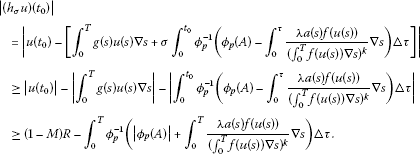(3.3)

From (H4), we have

$\begin{array}{rcl}|\left({h}_{\sigma }u\right)\left({t}_{0}\right)|& \ge & \left(1-M\right)R-{\int }_{0}^{T}{\varphi }_{p}^{-1}\left(|{\varphi }_{p}\left(A\right)|+\lambda {M}_{1}{\left({\int }_{0}^{T}f\left(u\left(s\right)\right)\mathrm{\nabla }s\right)}^{1-k}\right)\mathrm{△}\tau \\ \ge & \left(1-M\right)R-{\int }_{0}^{T}{\varphi }_{p}^{-1}\left[|{\varphi }_{p}\left(A\right)|+\lambda {M}_{1}{T}^{1-k}\left({c}_{1}{\varphi }_{p}\left(\parallel u\parallel \right)+{c}_{2}\right)\right]\mathrm{△}\tau \\ >& 0.\end{array}$
(3.4)

If $k>1$, choosing $R>\frac{{2}^{q-1}|A|T}{1-M-{2}^{q-1}{\varphi }_{p}^{-1}\left(\lambda {M}_{1}{T}^{1-k}{c}_{3}\right)T}$, then for any fixed $u\in \partial {B}_{R}\left(\theta \right)$, there exists a ${t}_{0}\in {\left[0,T\right]}_{\mathbb{T}}$ such that $|u\left({t}_{0}\right)|=R$. From (H5), we have

$\begin{array}{rcl}|\left({h}_{\sigma }u\right)\left({t}_{0}\right)|& \ge & \left(1-M\right)R-{\int }_{0}^{T}{\varphi }_{p}^{-1}\left(|{\varphi }_{p}\left(A\right)|+\frac{\lambda {M}_{1}}{{\left({\int }_{0}^{T}f\left(u\left(s\right)\right)\mathrm{\nabla }s\right)}^{k-1}}\right)\mathrm{△}\tau \\ \ge & \left(1-M\right)R-{\int }_{0}^{T}{\varphi }_{p}^{-1}\left[|{\varphi }_{p}\left(A\right)|+\lambda {M}_{1}{T}^{1-k}{c}_{3}{\varphi }_{p}\left(\parallel u\parallel \right)\right]\mathrm{△}\tau \\ >& 0.\end{array}$
(3.5)

If $k=1$, choosing $R>\frac{{\varphi }_{p}^{-1}\left(|{\varphi }_{p}\left(A\right)|+\lambda {M}_{1}\right)T}{1-M}$, then for any fixed $u\in \partial {B}_{R}\left(\theta \right)$, there exists a ${t}_{0}\in {\left[0,T\right]}_{\mathbb{T}}$ such that $|u\left({t}_{0}\right)|=R$. By direct calculation, we have

$\begin{array}{rcl}|\left({h}_{\sigma }u\right)\left({t}_{0}\right)|& \ge & \left(1-M\right)R-{\int }_{0}^{T}{\varphi }_{p}^{-1}\left(|{\varphi }_{p}\left(A\right)|+\lambda {M}_{1}\right)\mathrm{△}\tau \\ >& 0.\end{array}$
(3.6)

This implies ${h}_{\sigma }u\ne \theta$ and hence we obtain $\theta \notin {h}_{\sigma }\left(\partial {B}_{R}\left(\theta \right)\right)$.

Since $deg\left({h}_{1},{B}_{R}\left(\theta \right),\theta \right)=deg\left({h}_{0},{B}_{R}\left(\theta \right),\theta \right)=±1\ne 0$, we know that (3.2) admits a solution $u\in {B}_{R}\left(\theta \right)$, which implies that (1.1), (1.2) also admits a solution in ${B}_{R}\left(\theta \right)$. □

## References

1. Bebernes JW, Lacey AA: Global existence and finite-time blow-up for a class of non-local parabolic problems. Adv. Differ. Equ. 1997, 2: 927-953.

2. Bebernes JW, Li C, Talaga P: Single-point blow-up for non-local parabolic problems. Physica D 1999, 134: 48-60. 10.1016/S0167-2789(99)00057-3

3. Krzywicki A, Nadzieja T: Some results concerning the Poisson-Boltzmann equation. Zastos. Mat. 1991, 21(2):265-272.

4. Caglioti E, Lions P-L, Marchioro C, Pulvirenti M: A special class of stationary flows for two-dimensional Euler equations: a statistical mechanics description. Commun. Math. Phys. 1992, 143: 501-525. 10.1007/BF02099262

5. Wolansky G: A critical parabolic estimate and application to non-local equations arising in chemotaxis. Appl. Anal. 1997, 66: 291-321. 10.1080/00036819708840588

6. Kerefov AA: Nonlocal boundary value problems for parabolic equations. Differ. Uravn. 1979, 15: 74-78.

7. Vabishchevich PN: Nonlocal parabolic problems and the inverse heat-conduction problem. Differ. Uravn. 1981, 17: 1193-1199.

8. Gordeziani DG, Avalishvili G: Investigation of the nonlocal initial boundary value problems for some hyperbolic equations. Hiroshima Math. J. 2001, 31: 345-366.

9. Agarwal R, Bohner M, O’Regan D, Peterson A: Dynamic equations on time scales: a survey. J. Comput. Appl. Math. 2002, 141: 1-26. 10.1016/S0377-0427(01)00432-0

10. Atici FM, Guseinov GS: On Green’s functions and positive solutions for boundary value problems on time scales. J. Comput. Appl. Math. 2002, 141: 75-99. 10.1016/S0377-0427(01)00437-X

11. Bohner M, Peterson A: Advances in Dynamic Equations on Time Scales. Birkhäuser, Boston; 2003.

12. Hamal NA, Yoruk F: Positive solutions of nonlinear m -point boundary value problems on time scales. J. Comput. Appl. Math. 2009, 231: 92-105. 10.1016/j.cam.2009.02.003

13. Li WT, Sun HR: Positive solutions for second order m -point boundary value problems on time scales. Acta Math. Sin. Engl. Ser. 2006, 22(6):1797-1804. 10.1007/s10114-005-0748-5

14. Sidi Ammi MR, Torres DFM: Existence of positive solutions for non local p -Laplacian thermistor problems on time scales. J. Inequal. Pure Appl. Math. 2007., 8(3): Article ID 69

15. Sidi Ammi MR, Torres DFM: Existence of three positive solutions to some p -Laplacian boundary value problems. Discrete Dyn. Nat. Soc. 2012., 2012: Article ID 145050

16. Sidi Ammi MR, Torres DFM: Existence and uniqueness of a positive solution to generalized nonlocal thermistor problems with fractional order derivatives. Differ. Equ. Appl. 2012, 4: 267-276.

17. Wang DB: Existence multiplicity and infinite solvability of positive solutions for p -Laplacian dynamic equations on time scales. Electron. J. Differ. Equ. 2006., 2006: Article ID 96

18. Yang YT, Meng FW: Positive solutions of the singular semipositone boundary value problem on time scales. Math. Comput. Model. 2010, 52: 481-489. 10.1016/j.mcm.2010.03.045

## Acknowledgements

This work was supported by NSFC (11271154) and by Key Lab of Symbolic Computation and Knowledge Engineering of Ministry of Education and by the 985 program of Jilin University, and the first author is also supported by the Youth Studies Program of Jilin University of Finance and Economics (XJ2012006).

## Author information

Authors

### Corresponding author

Correspondence to Wenjie Gao.

### Competing interests

All authors declare that they have no competing interests.

### Authors’ contributions

WS dfafted this paper and WG checked and corrected the manuscript.

## Rights and permissions

Reprints and Permissions

Song, W., Gao, W. Existence of solutions for nonlocal p-Laplacian thermistor problems on time scales. Bound Value Probl 2013, 1 (2013). https://doi.org/10.1186/1687-2770-2013-1